## Coming soon: Addition and more fraction worksheetsNext week we have a little twist on the usual adding three numbers type of worksheet. On this page the third number is missing but the answer is given. What makes this harder is that at least two mental calculations have to be made to reach a correct answer. Quite a challenge for Year 2 children!
We also have a page showing the standard written method of addition.The method is to add the units first, put the units in the answer, and ‘carry’ the ten into the tens column. Finally add the tens and write in the total on the answer line.Plenty of practice with easy numbers.
Also coming soon is a great page for Year 5 on relating fractions and division. This fraction worksheet is a follow up to the page published last week, as children need plenty of practice with these ideas.

## Year 5 Maths Worksheet: relate fractions and division

Maths Worksheet: relate fractions and division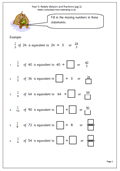The relationship between fractions and division is one which many children fail to grasp. Put simply, one fifth of 30 is equivalent to 30 divided by 5, or written as a fraction 30 over 5.

It can be a great help to see a fraction as a division calculation. 1/2 can also be thought of as one divided by two.

This page takes a quick look at this and should show whether your child does understand this important relationship.

## Resource of the Week: Solve puzzles and investigate

Investigations are a great way to reinforce mathematical concepts and learn about patterns in maths. This maths worksheet is posted in our Year 2 section, under Using and Applying Maths and has proved to be one of our most used resources.

We have the digits from one to nine again, this time the task is to use three digits to make 12. This is much harder than just using two digits and it would be a good idea to have some cards printed out with the digits on to help with this. They are available in the reception maths worksheets section.

Again plenty of opportunity for talking about the numbers and what counts as a different way. Is 1 + 2 + 9 the same as 2 + 1 + 9 ?

Free Y2 maths worksheet: Solve puzzles and investigate (2)

## Year 4 Maths Worksheet: division problems (pg 2)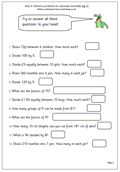This is a follow up page to the earlier solving division problems in your head worksheet. This has a range of different ways of asking division questions which children need to be able to recognise and there are a range of methods that can be used to work out the answers mentally.

It is important the tables are known so that the facts can be used. For example if it is known that 6 x 6 = 36, then 6 x 60 can be quickly worked out as 360, and in turn 360 divided by 6 is 60.

Another useful tip is when dividing by 5 mentally it is often possible to divide by 10 and then double the answer.

Division problems to calculate mentally (pg 2)

## Maths worksheet: Writing fractions in words or numbers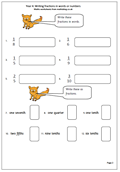Reading and writing fractions remain a bit of a mystery for many children, but our cunning foxes can help. This worksheet looks at how to read and write fractions from halves to tenths using numbers or words.  As well as reading a fraction such as 1/3 as one third, it can also be read as a division sum: 1 divided by 3, or 1 divided into 3 equal parts, but this comes a little later.

These pages can be found in our Year 4 section, under Counting and Numbers.

Writing fractions in words or numbers

## Coming soon: More fraction and division worksheets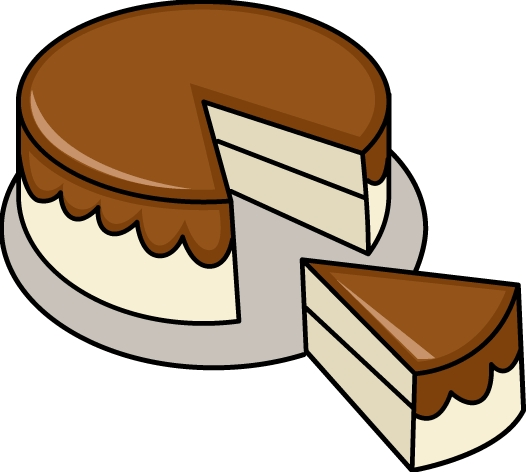Next week we solve one of the mysteries of fractions with a foxy worksheet which looks at how to read and write fractions from halves to tenths using numbers or words.

We also have a cunning page on division for year 4. This has a range of different ways of asking division questions which children need to be able to recognise and there are a number of methods that can be used to work out the answers mentally.

Continuing on the division and fraction theme we have a Year 5 worksheet which relates them both. It can be a great help to see a fraction as a division calculation. For example 1/6 can also be thought of as one divided by six or one whole one cut into six equal pieces.

## Year 2 maths worksheet: missing numbers on a number square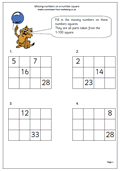This is the second page which looks in detail at a 1-100 number square and is excellent practice at understanding place value as well as counting.

A small section of the whole number square, just 4 by 3 is taken and most of the numbers removed. The skill is in replacing the missing numbers.

This can be done in a variety of ways. Probably the easiest way is to work across as each number is one more than the number to the left. One of the more interesting ways is to work down as each number is 10 more than the number above.

Missing numbers on a number square (pg 2)

## Resource of the Week: Written addition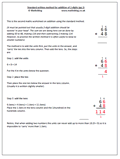We have a good selection of maths worksheets covering the standard written method of addition. We begin this with using just 2-digit numbers, although it should be pointed out that usually 2-digit addition should be tackled ‘in your head’.

The standard method is to add the units first, put the units in the answer, and ‘carry’ the ten into the tens column. Then add the tens.  detailed examples and plenty of preactice can be found in our Four Rules section under written addition.

Standard addition of 2-digit numbers (2)

## Year 5 Maths Worksheet: Solving division problems (pg 1)

Year 5 Maths Worksheet: solving division problems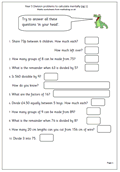By year 5 solving division problems ‘in your head’ get quite tricky and a very good understanding of the relationship between multiplication and division is needed. Questions involving sharing with remainders need to be solved which need both working out ‘tables’ and calculating the number left over.

It is always a good idea to ask children how they reached their answers as this can reveal a great deal about their mathematical thinking as well as their knowledge of ‘times tables’.

Here we have a page of division problems, all of which should be answered mentally, although ‘jottings’ may help.

Division problems to calculate mentally (pg 1)

## Year 4 maths worksheet: comparing fractions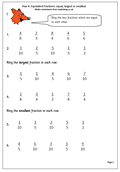Once the idea of equivalent fractions has been understood it becomes possible to compare two fractions to see which is the larger. The first thing to remember is that the larger the number on the bottom of the fraction, the smaller each part of the fraction is. So 4/100 is much smaller than 4/25.

Now this is also easy if the bottom number (denominator) is the same in each fraction eg 1/5 is smaller than 3/5.

The difficulty comes when the numbers are not the same. How do you compare 3/5 with 7/10?

The easiest way is to make the bottom number of each fraction the same, and in the case above this will mean converting the 3/5 into tenths. We can do this by multiplying the 5 by 2 to make 10 and we have to do the same with the top number, multiplying the 3 by 2. In this way 3/5 can be converted to 6/10.

3/5 and 6/10 are equal, or equivalent.

It is now easy to see that 6/10 is smaller than 7/10, so 3/5 must also be smaller than 7/10. Job done!

Later it will become harder to convert so that the denominators are the same; sometimes you have to multiply both fractions by different numbers, but this comes later! At the moment it is important to get the basics correct.

Equivalent fractions: comparing